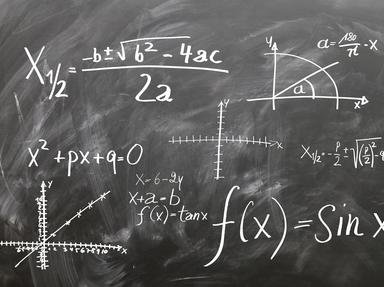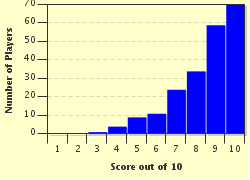# The Magic of Math Trivia Quiz

### Math is not my favorite subject, but I thought writing a quiz about it would help me understand it more.

A multiple-choice quiz by pennie1478. Estimated time: 3 mins.

Author
pennie1478
Time
3 mins
Type
Multiple Choice
Quiz #
384,115
Updated
Dec 03 21
# Qns
10
Difficulty
Easy
Avg Score
9 / 10
Plays
1372
Last 3 plays: Guest 157 (4/10), MikeyGee (9/10), Guest 24 (10/10).
This quiz has 2 formats: you can play it as a or as shown below.
Scroll down to the bottom for the answer key.
1. A fraction has a numerator and a denominator. On a fraction, what is the bottom number known as?

#### NEXT>

2. When working an equation, what is the first order of operation? Hint

Anything inside a parenthesis is worked first
Any multiplication and division problems are worked first
Working out exponents is completed first
Addition and subtraction problems are the first to be worked in an equation

#### NEXT>

3. What is the math term described as the likelihood that something will happen? Hint

Nonzero
Percentile
Probability
Logarithm

#### NEXT>

4. A triangle is a three sided figure. What kind of triangle has three different sides with three different angles, none of which is 90 degrees? Hint

Right triangle
Scalene triangle
Isosceles triangle
Equilateral triangle

#### NEXT>

5. A circle is a shape that has every point the same distance from the center. What is the distance, passing through the center, from one side of the circle to the other side of the circle? Hint

Diameter
Circumference
Arc

#### NEXT>

6. On a number line with zero in the center, all the numbers on the left of a horizontal number line would be negative numbers.

True
False

#### NEXT>

7. In this list of numbers, what would the median be: 196, 207, 219, 254, 265, 272, 280, 292, 300? Hint

300
265
196
280

#### NEXT>

8. In algebra, how is a variable most often represented? Hint

A parenthesis
A number
A letter
A dash

#### NEXT>

9. How many degrees are in a straight angle? Hint

360 degrees
45 degrees
90 degrees
180 degrees

#### NEXT>

10. Does the numerical value of pi go on forever?

Yes
No

 (Optional) Create a Free FunTrivia ID to save the points you are about to earn:Select a User ID:Choose a Password:Your Email:

Quiz Answer Key and Fun Facts
1. A fraction has a numerator and a denominator. On a fraction, what is the bottom number known as?

The numerator is the top number of a fraction. A line divides the numerator from the denominator. In a proper fraction, the numerator is smaller than the denominator. An improper fraction has a larger numerator than denominator. The mixed fraction is a whole number beside a fraction.
2. When working an equation, what is the first order of operation?

Answer: Anything inside a parenthesis is worked first

If the order of operations is not used in an equation, the answer will be different from the answer given when the order of operation is used. Anything in parenthesis should be taken care of first no matter what math is needed inside the parenthesis. After the problem inside the parenthesis is worked, deal with any exponents in the equation. Multiplication and division are third in the order of operation with addition and subtraction being the last thing done in an equation.
3. What is the math term described as the likelihood that something will happen?

A logarithm is the power that a number must be raised to in order to get another number. A nonzero number is any number other than zero. A percentile is the value in which a certain percentage falls.
4. A triangle is a three sided figure. What kind of triangle has three different sides with three different angles, none of which is 90 degrees?

A scalene triangle is a triangle with three different sides and three different angles.

A right triangle is a triangle with one angle that equals 90 degrees, and may also be classed as a scalene triangle.

An isosceles triangle is a triangle with two sides that are the same.

An equilateral triangle is a triangle with all three sides and all three angles equal.
5. A circle is a shape that has every point the same distance from the center. What is the distance, passing through the center, from one side of the circle to the other side of the circle?

The diameter is the distance from one side of a circle to the other side. The radius is half of a diameter. The circumference is the distance around the outside of the circle. An arc is part of the circumference.
6. On a number line with zero in the center, all the numbers on the left of a horizontal number line would be negative numbers.

On a number line, the numbers to the left of the zero are all negative numbers. All the numbers to the right of the zero on a number line are positive numbers, known as natural numbers.

A vertical axis can also be classed as a number line, when positive numbers go up and negative numbers go down.
7. In this list of numbers, what would the median be: 196, 207, 219, 254, 265, 272, 280, 292, 300?

To find the median in a list of numbers, first the numbers must be put into numerical order. If the list is even numbered, average the two middle numbers to find the median. If the list is odd numbered, the median would be the middle number.
8. In algebra, how is a variable most often represented?

A variable in algebra is an unknown number so it is usually represented by a letter of the alphabet, although other symbols can be used as well. In algebra, a constant is a number.
9. How many degrees are in a straight angle?

A straight angle has 180 degrees.
A reflex angle has degrees greater than 180 and less than 360 degrees.
A full rotation angle has 360 degrees.
An acute angle has less than 180 degrees.
A right angle has 90 degrees.
An obtuse angle is greater than 90 degrees and less than 180 degrees.
10. Does the numerical value of pi go on forever?

The absolute value of pi is not known because the decimal places go on forever. Pi to the twelfth place would be 3.141592653589.
Source: Author pennie1478

This quiz was reviewed by FunTrivia editor rossian before going online.
Any errors found in FunTrivia content are routinely corrected through our feedback system.
Most Recent Scores
Jan 21 2023 : Guest 157: 4/10
Jan 21 2023 : MikeyGee: 9/10
Jan 20 2023 : Guest 24: 10/10
Jan 06 2023 : Guest 73: 9/10
Dec 30 2022 : Guest 98: 7/10
Dec 30 2022 : hosertodd: 9/10
Dec 15 2022 : Guest 104: 10/10

Score DistributionRelated Quizzes
This quiz is part of series Be Afraid, Be Very Afraid:

A quiz list all who fear multiplying or dividing by six should definitely avoid. For those not afraid of simple math, enjoy!

1. The Magic of Math Easier
2. Elementary Math Average
3. Simple Statistics Average
4. Mixed Number Skills Easier
5. Square Roots Very Easy